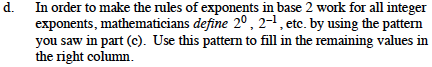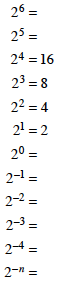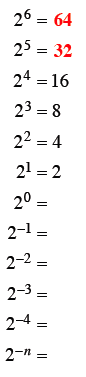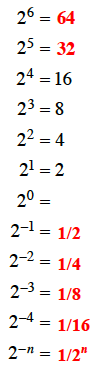### Home > PC > Chapter 1 > Lesson 1.1.1 > Problem1-8

1-8.
1.  26 = 25 = 24 = 16 23 = 8 22 = 4 21 = 2 20 = 2−1 = 2−2 = 2−3 = 2−4 = 2−n =

REVIEW OF ZERO AND NEGATIVE EXPONENTS

Sarah was teaching her younger sister Tanya about exponents. Tanya thought that 20 = 0 and 2–1 = –2, but Sarah told her that was wrong. To help Tanya, Sarah made a table like the one shown at right and filled in the values shown. Homework Help ✎

1. Sarah told Tanya that the numbers in the right column double each time as you go up. Explain why they are double by writing 22, 23, and 24 as products of 2’s.

2. On your own paper, copy the table and fill in the values for 25 and 26.

3. What happens to the values in the right column as we go down from 26?

4. In order to make the rules of exponents in base 2 work for all integer exponents, mathematicians define 20, 2−1, etc. by using the pattern you saw in part (c). Use this pattern to fill in the remaining values in the right column.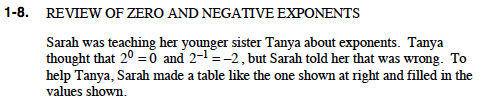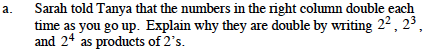22 = 2·2
23 = 2·2·2
24 = 2·2·2·2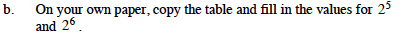They are reduced by half.